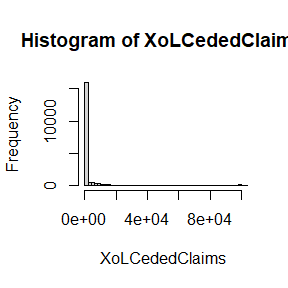# SlicedDistributions

## 1.Introduction

This vignette will cover the sliced distributions available in the NetSimR package. It will overview the theory behind these functions, propose posible uses and illustrate examples. Currently, Sliced distributions available are LogNormal and Gamma with a Pareto distribution for the tail.

## 2.Backround theory

### 2.1 Sliced distribution backround

At various insurance modelling exercises, it may be the case that a sliced distribution provides a significantly better fit than a non-sliced distribution. Tail losses may be very influential on the premium, as such modelling the tail more accurately would significantly improve the accuacy of the premium. This becomes more important when modelling upper layers that will be mainly based on the severity tail. The analyst may opt to model attritional losses using a regression and have a single dimensional Pareto covering all cases. Theory suggests that Pareto distributions provide an appropriate fit for the tail of distributions and the mean excess function can be used to set the slice point. The analyst may consider using a truncated or censored distribution fit for the attritional data, in order to improve the fit.

### 2.2 Sliced distribution definition

Let claim severity $$x$$ have a probability density function $$f(x)$$ and a cumulative density funtion $$F(x)$$. Let $$s$$ be the slice point. We define $$f(x)$$ as: $f(x)= \left[\begin{array} {rrr} g(x) & x \le s \\ (1-G(s))*h(x) & x > s \end{array}\right]$ $$g(x)$$ is the attritional attritional probability density function, fitted to the full claims. As suggested this could be fitted to full claims as a censored distribution or to the claims below $$s$$ as a truncated distribution for a better fit. $$g(x)$$ in NetSimR package will either be Gamma or LogNormal. $$h(x)$$ is the large probability density function. $$h(x)$$ in NetSimR package will be a single parameter Pareto distribution. The scale paramter $$x_{m}$$ will be the slice point $$s$$. The shape parameter will be estimated from the claims larger than the slice point. $$H(x)$$ and $$G(x)$$ are the cumulative density functions of $$h(x)$$ and $$g(x)$$ respectively.

We define $$F(x)$$ as: $F(x)= \left[\begin{array} {rrr} G(x) & x \le s \\ G(s)+(1-G(s))*H(x) & x > s \end{array}\right]$ The mean $$E(x)$$ is defined to be: $E(x)=Capped Mean (g(x) @ s) + (1-G(s))*(Mean(h(x))-s)$ Let claims be capped at amount $$c$$. The capped claim severity is defined as $$y=Min(x,c)$$.The capped mean $$E(y)$$ is defined to be: $E(y)= \left[\begin{array} {rrr} Capped Mean (g(y)@c) & c \le s \\ Capped Mean (g(x) @ s)+(1-G(s))*(Capped Mean (h(y)@c)-s) & c > s \end{array}\right]$

## 3. Functions usage

NetSimR package provides functions for the mean, capped mean, exposure curve and increased limit factor curves. These were discussed in the “Capped mean, Exposure and Increased Limit Factor curves” vignette and will not be repeated here. The package also provides the probability density function (pdf), the cumulative probability density function (cdf) and the inverse cumulative probability function for the sliced distributions.

The probability density function can be used for optimising parameters with the maximum likelihood method, when fitting such a distribution, in cases where fitting the distributions separately is not appropriate.

The cumulative density function provides probability of claims being less or more than a particular level. The distribution can be used alongst methods such as the Panjer recursion, in order to combine it with the frequency distribution and provide the aggrecate claims distribution.

The inverse cumulative probability distribution can be used to run simulations over the defined distribution. Reinsurance strucutures can then be applied to use the simulation exercise for reinsurance pricing.

## 4. Examples

### 4.1 Mean of a sliced distribution

Here we calculate the expected severity from a sliced lognormal pareto distributions, sliced at 10,000 currency units, with a Pareto shape parameter $$shape = 1.2$$ and lognormal parameters $$mu = 6$$ and $$sigma = 1.6$$.

library("NetSimR")
round(SlicedLNormParetoMean(6,1.6,10000,1.2),0)
##  2299

Multiplying the above with claim frequency would provide the expected claims cost. A capped mean of a sliced distribution follows the capped mean vignette examples.

### 4.2 Sliced distribution simulations

We will only demonstrate simulating from the inverse distribution, the probability density and cumulative probability functions’ use is simpler. Below we simulate from a sliced distribution, applying an each and every loss excess of loss reinsurance structure.

#set seed
set.seed(1)

#set parameters
numberOfSimulations=20000
freq=15
mu=6
sigma=1.6
s=1000
alpha=1.2
Deductible=10000
limit=100000
XoLCededClaims <- numeric(length = numberOfSimulations)

#loop simulates frequency then severity and applies layer deductible and limit
for (i in 1 :numberOfSimulations){
x=qSlicedLNormPareto(runif(rpois(1,freq)),mu,sigma,s,alpha)
x=ifelse(x<Deductible,0,ifelse(x-Deductible>limit,limit,x-Deductible))
XoLCededClaims[i]=round(sum(x),0)
}

#Visualising Simulation results
head(XoLCededClaims)
##      0  9463     0     0     0 11173
hist(XoLCededClaims, breaks = 100, xlim = c(0,100000))summary(XoLCededClaims)
##    Min. 1st Qu.  Median    Mean 3rd Qu.    Max.
##       0       0       0    5300       0  201873